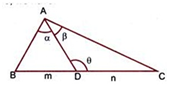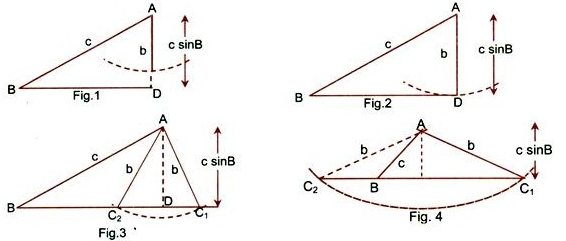×#### Thank you for registering.

One of our academic counsellors will contact you within 1 working day.

Click to Chat

1800-1023-196

+91-120-4616500

CART 0

• 0

MY CART (5)

Use Coupon: CART20 and get 20% off on all online Study Material

ITEM
DETAILS
MRP
DISCOUNT
FINAL PRICE
Total Price: Rs.

There are no items in this cart.
Continue Shopping• Complete JEE Main/Advanced Course and Test Series
• OFFERED PRICE: Rs. 15,900
• View Details

```Revision Notes on Solution of Triangles

Sine rule: Sides of a triangle are proportional to the sine of the angles opposite to them. So, in ΔABC,

sin A/a = sin B/b = sin C/c = 2Δ/abc.

This may also be written as (a/sin A) = (b/sin B) = (c/sin C)

Cosine rule: In any ΔABC,

cos A = (b2 + c2 – a2) /2bc

cos B = (a2 + c2 – b2)/2ac

cos C = (a2 + b2 - c2)/2ab

Trigonometric ratios of half-angles:

sin A/2 = √[(s-b)(s-c)/bc]

sin B/2 = √[(s-c) (s-a)/ac]

sin C/2 = √[(s-a) (s-b)/ab]

cos A/2 = √s(s - a)/bc

cos B/2 = √s(s - b)/ac

cos C/2 = √s(s - c)/ab

tan A/2 = √[(s - b) (s - c)/s(s - a)]

tan B/2 = √[(s - c) (s - a)/s(s - b)]

tan C/2 = √[(s - a) (s - b)/s(s - c)]

Projection rule: In any ΔABC,

a = b cos C + c cos B

b = c cos A + a cos C

c = a cos B + b cos A

Area of a triangle

If Δ denotes the area of the triangle ABC, then it can be calculated in any of the following forms:

Δ = 1/2 bc sin A = 1/2 ca sin B = 1/2 ab sin C

Δ = √s(s - a)(s – b)(s - c)

Δ = 1/2. (a2 sin B sin C)/ sin(B + C)

= 1/2. (b2 sin C sin A)/ sin (C + A)

= 1/2. (c2 sin A sin B)/ sin (A + B)

Semi-perimeter of the triangle

If S denotes the perimeter of the triangle ABC, then s = (a + b + c)/2

Napier’s analogy

In any ΔABC,

tan [(B – C)/2] = (b – c)/(b + c) cot A/2

tan [(C – A) /2] = (c – a)/(c + a) cot B/2

tan [(A – B) /2] = (a – b)/(a + b) cot C/2

m-n theorem

Consider a triangle ABC where D is a point on side BC such that it divides the side BC in the ratio m: n, then as shown in the figure, the following results hold good:(m + n) cot θ = m cot α – n cot ß.

(m + n) cot θ = n cot B – m cot C.

Apollonius theorem

In a triangle ABC, if AD is the median through A, then

AB2 + AC2 = 2(AD2 + BD2).

If the three sides say a, b and c of a triangle are given, then angle A is obtained with the help of the formula

tan A/2 = √(s - b) (s - c) / s(s - a) or cos A = b2 + c2 - a2 / 2bc.

Angles B and C can also be obtained in the same way.

If two sides b and c and the included angle A are given, then

tan (B – C) /2 = (b – c)/ (b + c) cot A/2

This gives the value of (B- C)/2.

Hence, using (B + C)/2 = 90o - A/2 along with the last equation both B and C can be evaluated. Now, the sides can be evaluated using the formula

a = b sin A/sin B or a2 = b2 + c2 – 2bc cosA.

If two sides b and c and the angle B (opposite to side b) are given, then using the following results, we can easily obtain the remaining elements

sin C = c/b sinB, A = 180o – (B + C) and b = b sin A/sinB

Some of the important points which must be remembered include:

b < c sin B, there is no triangle possible (Fig. 1)

If b = c sin B and B is an acute angle, then only one triangle is possible (Fig. 2)

If c sin B < b < c and B is an acute angle, then there are two values of angle C (Fig. 3).

If c < b and B is an acute angle, then there is only one triangle (Fig. 4).```### Course Features

• 728 Video Lectures
• Revision Notes
• Previous Year Papers
• Mind Map
• Study Planner
• NCERT Solutions
• Discussion Forum
• Test paper with Video Solution